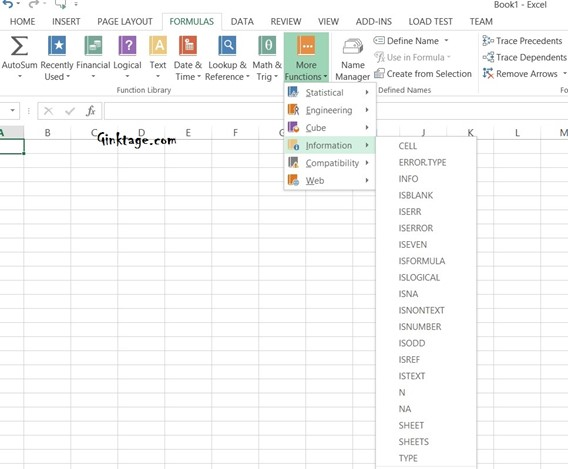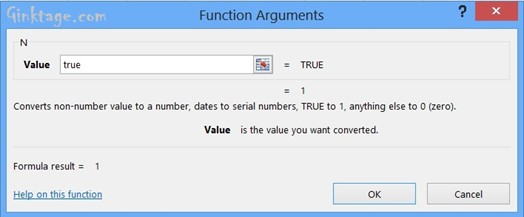# How to Convert Non-Numeric value to Numeric in Microsoft Excel 2013?

This article will explain how to convert a simple alphanumeric value to numeric value in Microsoft Excel 2013.

There are times when the users may need to convert a non-numeric value (for example Yes or No) to its Numeric value when using Microsoft Excel Spreadsheet. Microsoft Excel 2013 offers an easy way to evaluate an Non numeric value using simple formula and display its numeric equivalent.

# How to Convert Non-Numeric value to Numeric in Microsoft Excel 2013?

To translate the non-numeric value to numeric value in Microsoft Excel, follow the below steps

1. Launch Microsoft Excel 2013 and create a blank workbook or open an existing workbook.

2. Navigate to the Formula’s tab and from the “More Functions” dropdown button, select, Information -> “N”.3. This will display the Functions Argument Dialog. Enter the non-numeric value. For example – TRUE. This will display numeric the value of true.